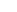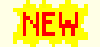# Aapo Hyvärinen: Publications

## Publications by topic## Bayesian networks, causal analysis, structural equation models

[Here we propose a new identifiable framework for estimation/learning of continuous-valued Bayesian networks or structural equation models, with application in causal analysis. The framework has linear and non-linear variants.]

#### Linear models

S. Shimizu, P.O. Hoyer, A. Hyvärinen, and A. Kerminen. A Linear Non-Gaussian Acyclic Model for Causal Discovery. J. of Machine Learning Research 7:2003-2030, 2006.
pdf    matlab/octave code Python code R code
[Proposes a new framework, "LiNGAM", for structural equation modelling, continuous-valued Bayesian networks, and causal inference based on non-gaussianity. Based on UAI2005 paper.]R. Pio Monti and A. Hyvärinen. A Unified Probabilistic Model for Learning Latent Factors and Their Connectivities from High-Dimensional Data. UAI2018.
pdf
[Proposes a framework combining dimension reduction by grouping of variables with causal discovery. The variable grouping is shown to be well-defined (identifiable). This allows for LiNGAM and related methods to be used in very high-dimensional data.]

A. Hyvärinen and S. M. Smith. Pairwise Likelihood Ratios for Estimation of Non-Gaussian Structural Equation Models. J. of Machine Learning Research 14:111-152, 2013.
pdf Matlab code
[Proposes new estimators for the LiNGAM model of the preceding paper. These estimators have simple intuitive interpretations and are statistically better at least in some circumstances. Earlier version was in ACML2010.]

S. Shimizu, T. Inazumi, Y. Sogawa, A. Hyvärinen, Y. Kawahara, T. Washio, P. O. Hoyer and K. Bollen. DirectLiNGAM: A direct method for learning a linear non-Gaussian structural equation model. J. of Machine Learning Research 12:1225-1248, 2011.
pdf matlab code
[A new algorithm for estimating the model proposed in the JMLR2006 paper. Based on our UAI 2009 paper.]

Y. Sogawa, S. Shimizu, A. Hyvärinen, T. Washio, T. Shimamura and S. Imoto. Estimating Exogenous Variables in Data with More Variables than Observations.. Neural Networks, 24:875--880, 2011.
pdf
[Considers causal discovery using non-gaussianity in the case where the number of variables is very large.]

A. Hyvärinen, K. Zhang, S. Shimizu, and P.O. Hoyer. Estimation of a Structural Vector Autoregression Model Using Non-Gaussianity J. of Machine Learning Research, 11:1709-1731, 2010.
pdf   Videolecture   R code
[Shows how to combine classic autoregressive modelling with instantaneous Bayesian networks (LiNGAM). Based on ICML2008 and ECML2009 papers.]

P.O. Hoyer, A. Hyvärinen, R. Scheines, P. Spirtes, J. Ramsey, G. Lacerda, and S. Shimizu. Causal discovery of linear acyclic models with arbitrary distributions. Conf. on Uncertainty in Artificial Intelligence (UAI2008), Helsinki, Finland.
pdf
[Extends the LiNGAM approach to the case where some of the variables can be gaussian.]

S. Shimizu, P.O. Hoyer, and A. Hyvärinen Estimation of linear non-Gaussian acyclic models for latent factors. Neurocomputing, 72:2024-2027, 2009.
pdf
[Extends the LiNGAM framework to causal connections between latent factors.]

S. Shimizu, A. Hyvärinen Discovery of linear non-gaussian acyclic models in the presence of latent classes. Proc. Int. Conf. on Neural Information Processing (ICONIP2007), pp. 752--761, 2008.
pdf
[Extends the LiNGAM framework to the case of latent classes.]

S. Shimizu, A. Hyvärinen, P.O. Hoyer, and Y. Kano. Finding a causal ordering via independent component analysis. Computational Statistics & Data Analysis, 50(11):3278-3293, 2006.
pdf
[Shows how a causal ordering can be estimated for non-gaussian variables based on ICA. An earlier version of the LiNGAM framework.]

#### Non-linear modelsR. Pio Monti, K. Zhang, and A. Hyvärinen. Causal Discovery with General Non-Linear Relationships Using Non-Linear ICA. UAI2019.
pdf
[A nonlinear version of LiNGAM. That is, causal discovery when the relationships (structural equations) have arbitrary nonlinear shapes. Made possible by our recent advances in nonlinear ICA]

K. Zhang and A. Hyvärinen. Nonlinear Functional Causal Models for Distinguishing Cause from Effect. In Statistical and Causality.
Wiley, 2016.
pdf
[A tutorial introduction to causal discovery with nonlinear models.]

K. Zhang and A. Hyvärinen. On the Identifiability of the Post-Nonlinear Causal Model. UAI 2009.
pdf
[Analyzes further the identifiability of the nonlinear model of NIP2008 workshop paper below. Related to the identifiability on post-nonlinear ICA, for which we obtain somewhat surprising results.]

K. Zhang and A. Hyvärinen. Causality Discovery with Additive Disturbances: An Information-Theoretical Perspective. ECML 2009.
pdf
[Provides a general theoretical framework for the LiNGAM model, and applies it on nonlinear and time-dependent models.]

K. Zhang and A. Hyvärinen. Distinguishing Causes from Effect using Nonlinear Acyclic Causal Models. JMLR Workshop and Conference Proceedings. Causality: Objectives and Assessment (NIPS 2008), 6:157-164, 2010.
pdf
[Develops a strongly nonlinear version of the LiNGAM framework introduced above. See UAI2009 paper for more recent results.]

J. Hirayama and A. Hyvärinen. Structural equations and divisive normalization for energy-dependent component analysis. Advances in Neural Information Processing (NIPS2011), Granada, Spain, 2012.
pdf
[Proposes a version of SEM where the interactions happen between the energies of the components.]

K. Zhang and A. Hyvärinen. Source separation and higher-order causal analysis of MEG and EEG. UAI2010.
pdf
[Develops a model of causal relations of variances of time signals.]